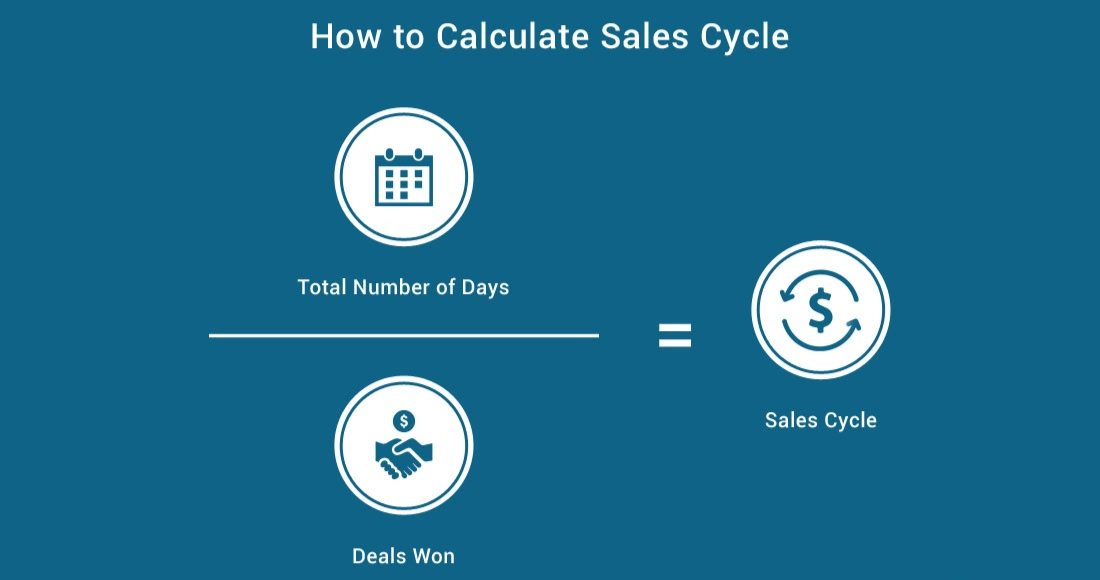# How to Calculate Sales Cycle

Sep 18th, 2018This blog post was originally published by Rekener, now a Brainshark company.

Sales cycle measures the average amount of time between when an opportunity or deal is created, and when it is closed won.

To calculate sales cycle, you first isolate all the deals that you won in a period. Then, for each one, you track the number of days between when it was created and when it was closed.  You sum up all those days from all the opps, and then divide by the number of opps won.

For example, if you had 50 deals that were won in Q2, and the total number of days from creation to closed won for all of them combined was 1,250, then your sales cycle would be 1,250 / 50 = 25 days.

To measure sales cycle with Salesforce data, you need to run a report of opportunities won in the period you want to measure.  Count how many opps that is. Then, for each opp, calculate the number of days between Created Date and Close Date. Sum up that total number of days.  Then divide the number of days by number of opps you won.

To measure sales cycle with HubSpot CRM data, you need to run a report of deals won in the period you want to measure.  Count how many deals that is. Then, for each deal, calculate the number of days between Created Date and Close Date. Sum up that total number of days. Then divide the number of days by number of deals you won.

Sales scorecards can automate sales cycle calculations, and can break conversion rates down by sales rep, opportunity type, industry, product, or any other dimension.  Check out our sales scorecard solution to see how we help calculate sales cycle by sales rep automatically.

Looking for more sales metrics know-how? Our comprehensive Sales Metrics Glossary will show you how to calculate 30 critical KPIs using CRM data.